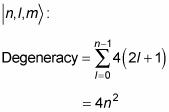##### Quantum Physics For Dummies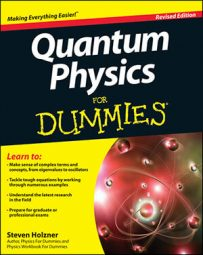When calculating the spin of an electron in a hydrogen atom, you need to allow for the spin of the electron, which provides additional quantum states. Given the following equation, where the wave function of the hydrogen atom is a product of radial and angular parts,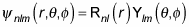you can add a spin part, corresponding to the spin of the electron, where s is the spin of the electron and ms is the z component of the spin: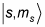The spin part of the equation can take the following values: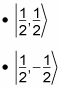Hence,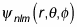now becomes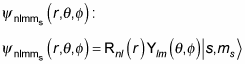And this wave function can take two different forms, depending on ms, like this: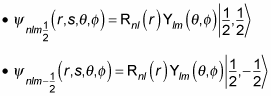In fact, you can use the spin notation, where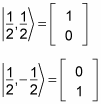For example, for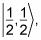you can write the wave function as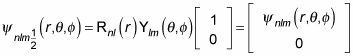And for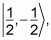you can write the wave function as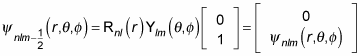What does this do to the energy degeneracy? If you include the spin of the electron, there are two spin states for every state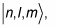so the degeneracy becomes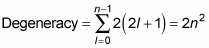So if you include the electron’s spin, the energy degeneracy of the hydrogen atom is 2n2.

In fact, you can even add the spin of the proton to the wave function (although people don’t usually do that, because the proton’s spin interacts only weakly with magnetic fields applied to the hydrogen atom). In that case, you have a wave function that looks like the following: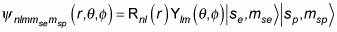where se is the spin of the electron, mse is the z component of the electron’s spin, sp is the spin of the proton, and msp is the z component of the proton’s spin.

If you include the proton’s spin, the wave function can now take four different forms, depending on ms, like this: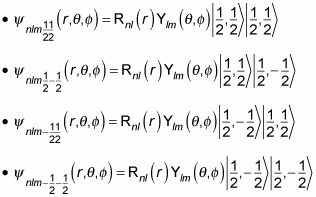The degeneracy must now include the proton’s spin, so that’s a factor of four for each